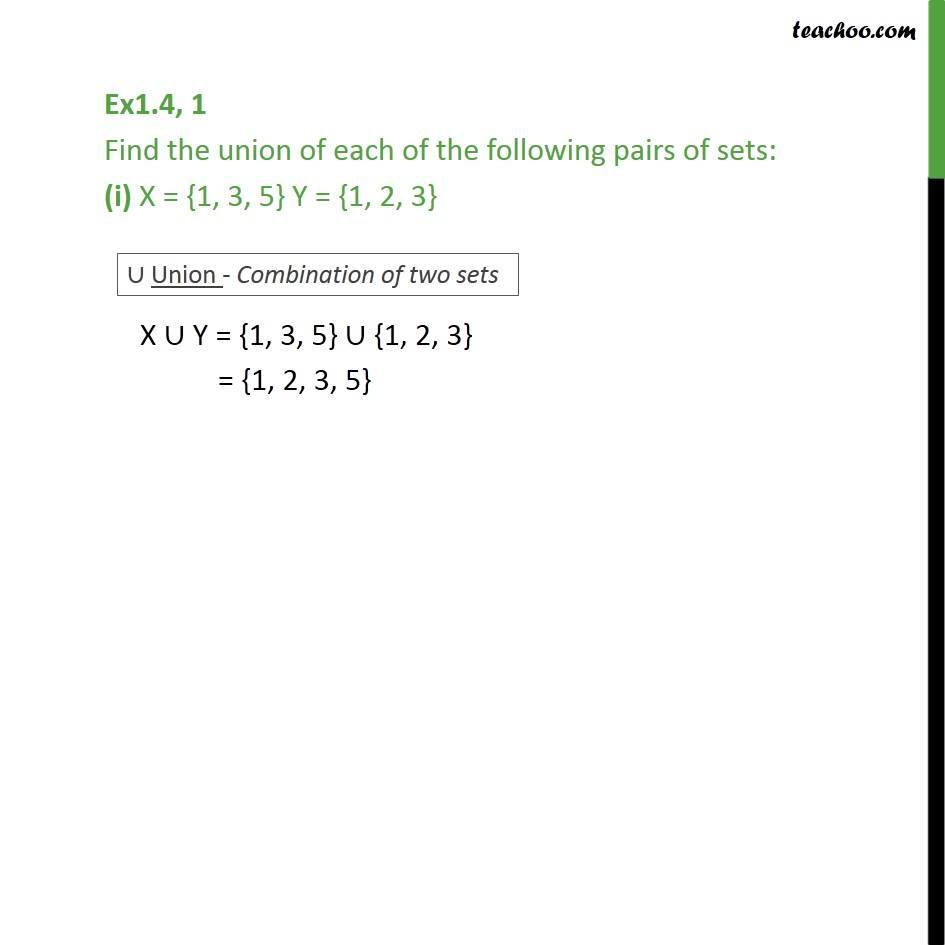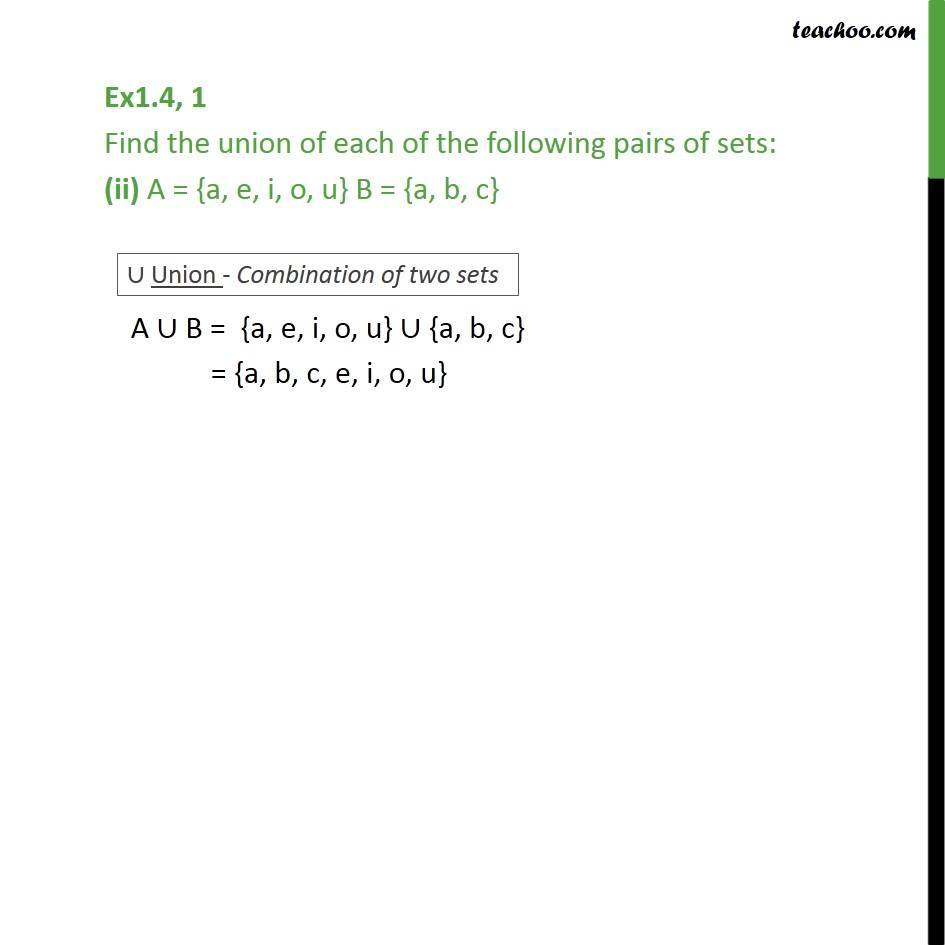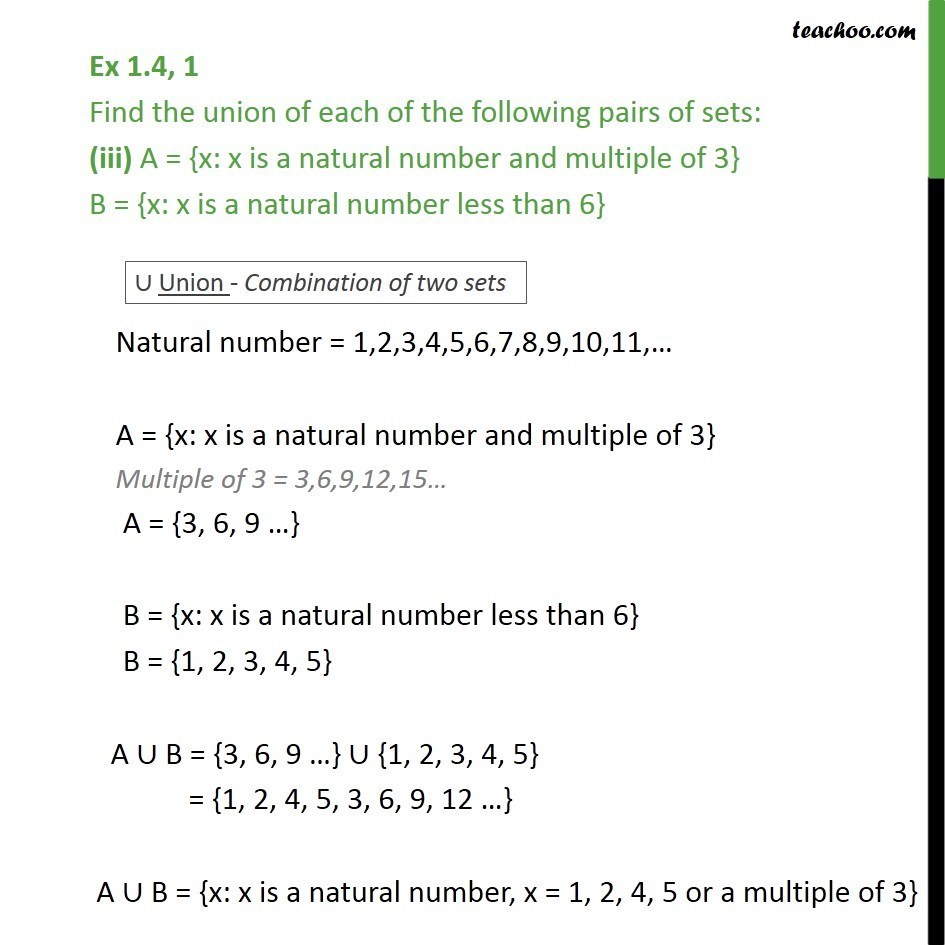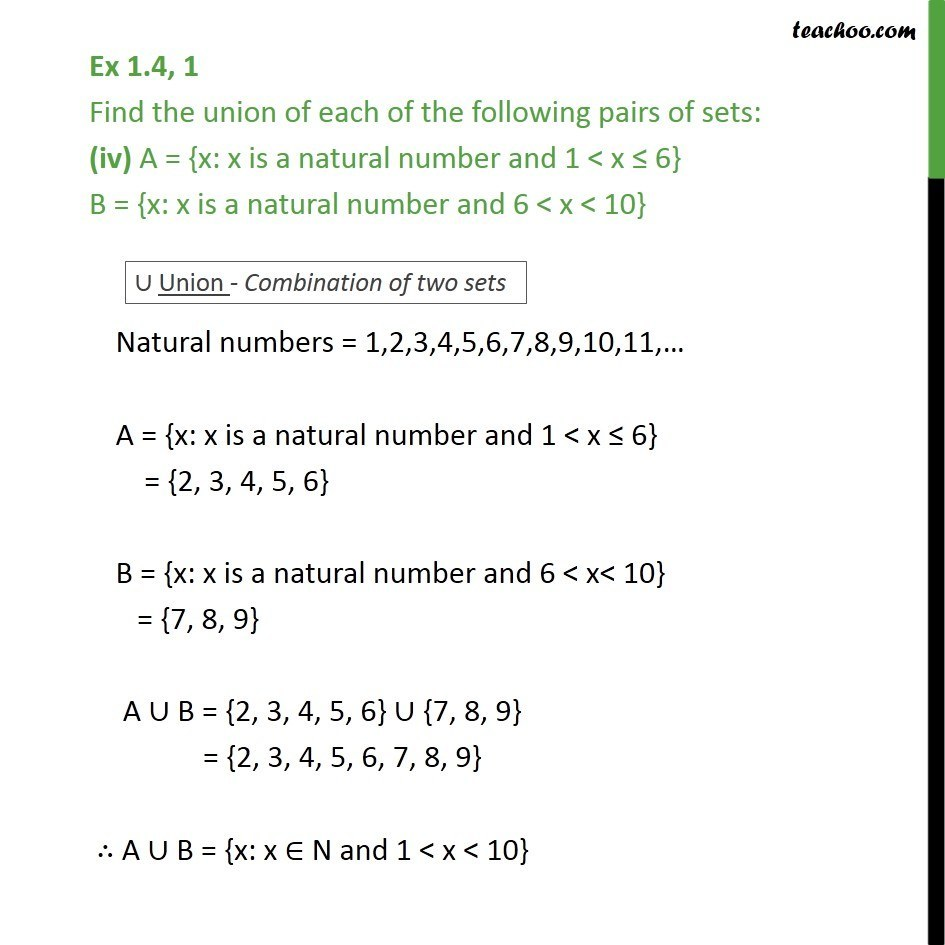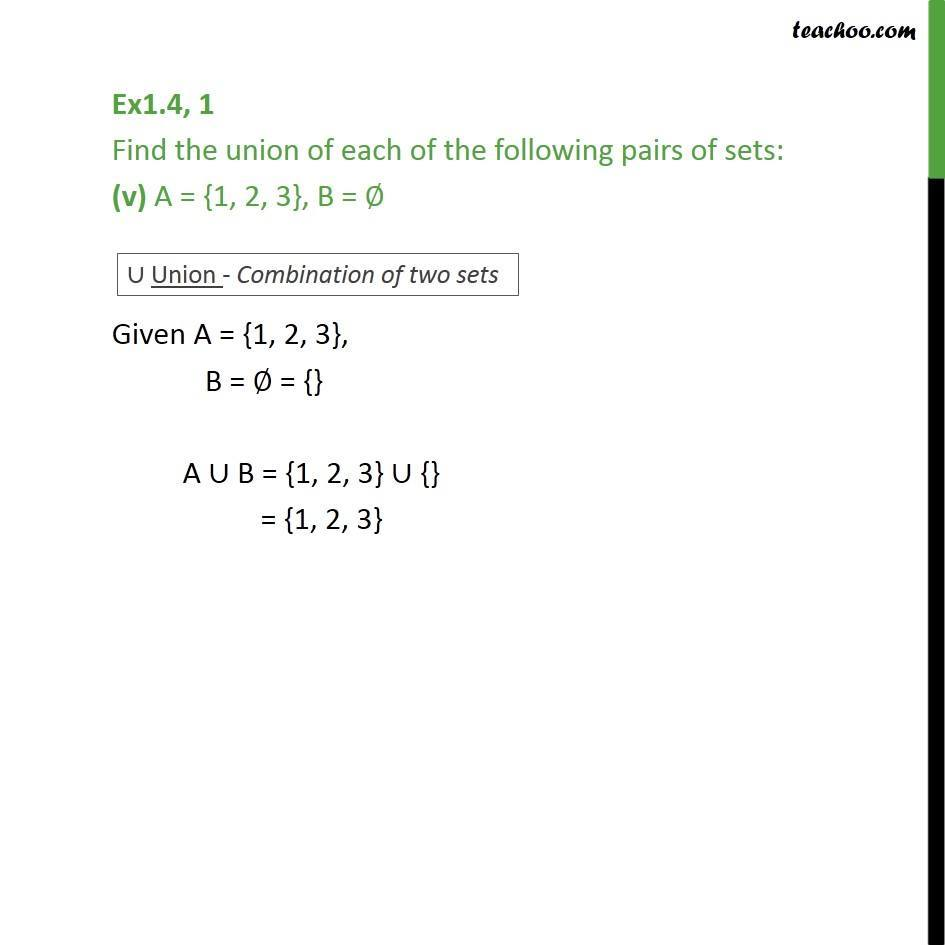1. Chapter 1 Class 11 Sets
2. Concept wise
3. Venn Diagram and Union of Set

Transcript

Ex1.4, 1 Find the union of each of the following pairs of sets: (i) X = {1, 3, 5} Y = {1, 2, 3} X Y = {1, 3, 5} {1, 2, 3} = {1, 2, 3, 5} Ex1.4, 1 Find the union of each of the following pairs of sets: (ii) A = {a, e, i, o, u} B = {a, b, c} A B = {a, e, i, o, u} {a, b, c} = {a, b, c, e, i, o, u} Ex 1.4, 1 Find the union of each of the following pairs of sets: (iii) A = {x: x is a natural number and multiple of 3} B = {x: x is a natural number less than 6} Natural number = 1,2,3,4,5,6,7,8,9,10,11, A = {x: x is a natural number and multiple of 3} Multiple of 3 = 3,6,9,12,15 A = {3, 6, 9 } B = {x: x is a natural number less than 6} B = {1, 2, 3, 4, 5} A B = {3, 6, 9 } {1, 2, 3, 4, 5} = {1, 2, 4, 5, 3, 6, 9, 12 } A B = {x: x is a natural number, x = 1, 2, 4, 5 or a multiple of 3} Ex 1.4, 1 Find the union of each of the following pairs of sets: (iv) A = {x: x is a natural number and 1 < x 6} B = {x: x is a natural number and 6 < x < 10} Natural numbers = 1,2,3,4,5,6,7,8,9,10,11, A = {x: x is a natural number and 1 < x 6} = {2, 3, 4, 5, 6} B = {x: x is a natural number and 6 < x< 10} = {7, 8, 9} A B = {2, 3, 4, 5, 6} {7, 8, 9} = {2, 3, 4, 5, 6, 7, 8, 9} A B = {x: x N and 1 < x < 10} Ex1.4, 1 Find the union of each of the following pairs of sets: (v) A = {1, 2, 3}, B = Given A = {1, 2, 3}, B = = {} A B = {1, 2, 3} {} = {1, 2, 3}

Venn Diagram and Union of Set

About the AuthorDavneet Singh
Davneet Singh is a graduate from Indian Institute of Technology, Kanpur. He has been teaching from the past 9 years. He provides courses for Maths and Science at Teachoo.